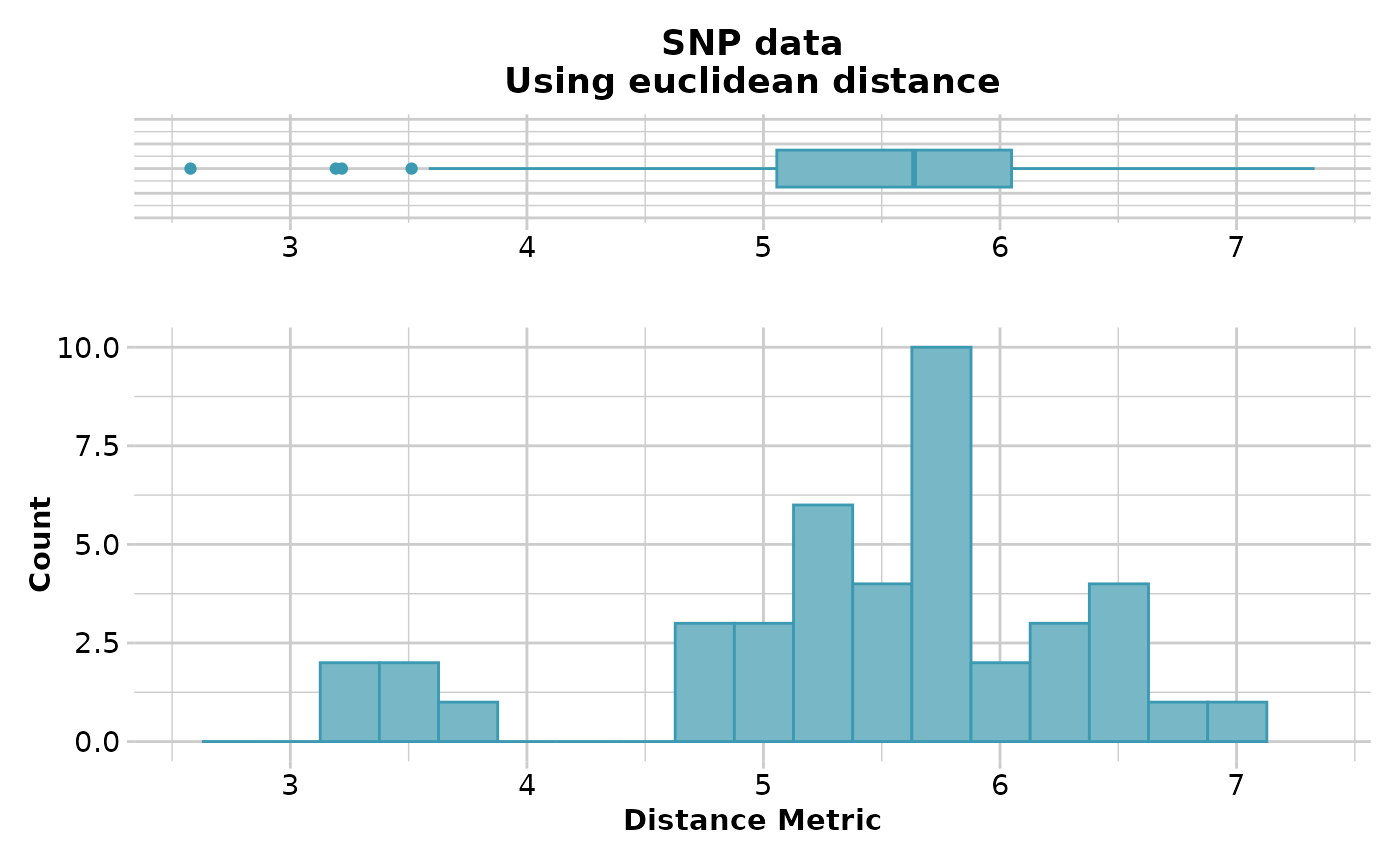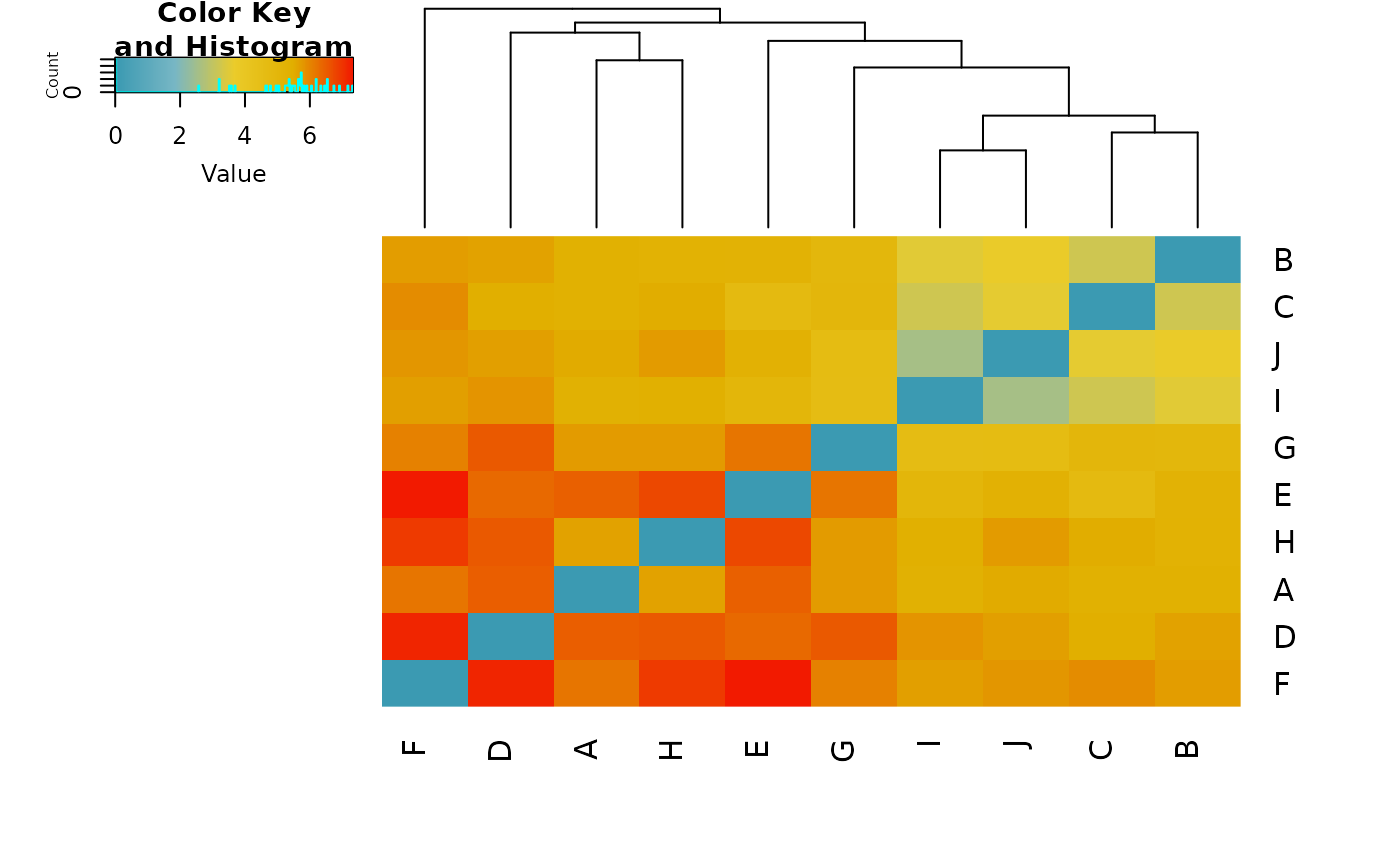The script plots a heat map to represent the distances in the distance or dissimilarity matrix. This function is a wrapper for heatmap.2 (package gplots).

## Usage

gl.plot.heatmap(D, palette_divergent = diverging_palette, verbose = NULL, ...)

## Arguments

D

Name of the distance matrix or class fd object [required].

palette_divergent

A divergent palette for the distance values [default diverging_palette].

verbose

Verbosity: 0, silent or fatal errors; 1, begin and end; 2, progress log; 3, progress and results summary; 5, full report [default 2 or as specified using gl.set.verbosity]

...

Parameters passed to function heatmap.2 (package gplots)

## Author

Custodian: Luis Mijangos -- Post to https://groups.google.com/d/forum/dartr)

## Examples

if (FALSE) {
gl <- testset.gl[1:10,]
D <- dist(as.matrix(gl),upper=TRUE,diag=TRUE)
gl.plot.heatmap(D)
D2 <- gl.dist.pop(possums.gl)
gl.plot.heatmap(D2)
D3 <- gl.fixed.diff(testset.gl)
gl.plot.heatmap(D3)
}
if ((requireNamespace("gplots", quietly = TRUE))) {
D2 <- gl.dist.pop(possums.gl)
gl.plot.heatmap(D2)
}
#> Starting gl.dist.pop
#>   Processing genlight object with SNP data
#>   Calculating distances:  euclidean
#>   Refer to the dartR Distance Analysis tutorial for algorithms
#>
#>   Returning a stats::dist object
#> Completed: gl.dist.pop
#> Starting gl.plot.heatmap
#>
#> Found more than one class "dist" in cache; using the first, from namespace 'spam'
#> Also defined by ‘BiocGenerics’
#> Found more than one class "dist" in cache; using the first, from namespace 'spam'
#> Also defined by ‘BiocGenerics’
#> Found more than one class "dist" in cache; using the first, from namespace 'spam'
#> Also defined by ‘BiocGenerics’
#> Found more than one class "dist" in cache; using the first, from namespace 'spam'
#> Also defined by ‘BiocGenerics’#>   Processing a distance matrix
#>#> Completed: gl.plot.heatmap
#>